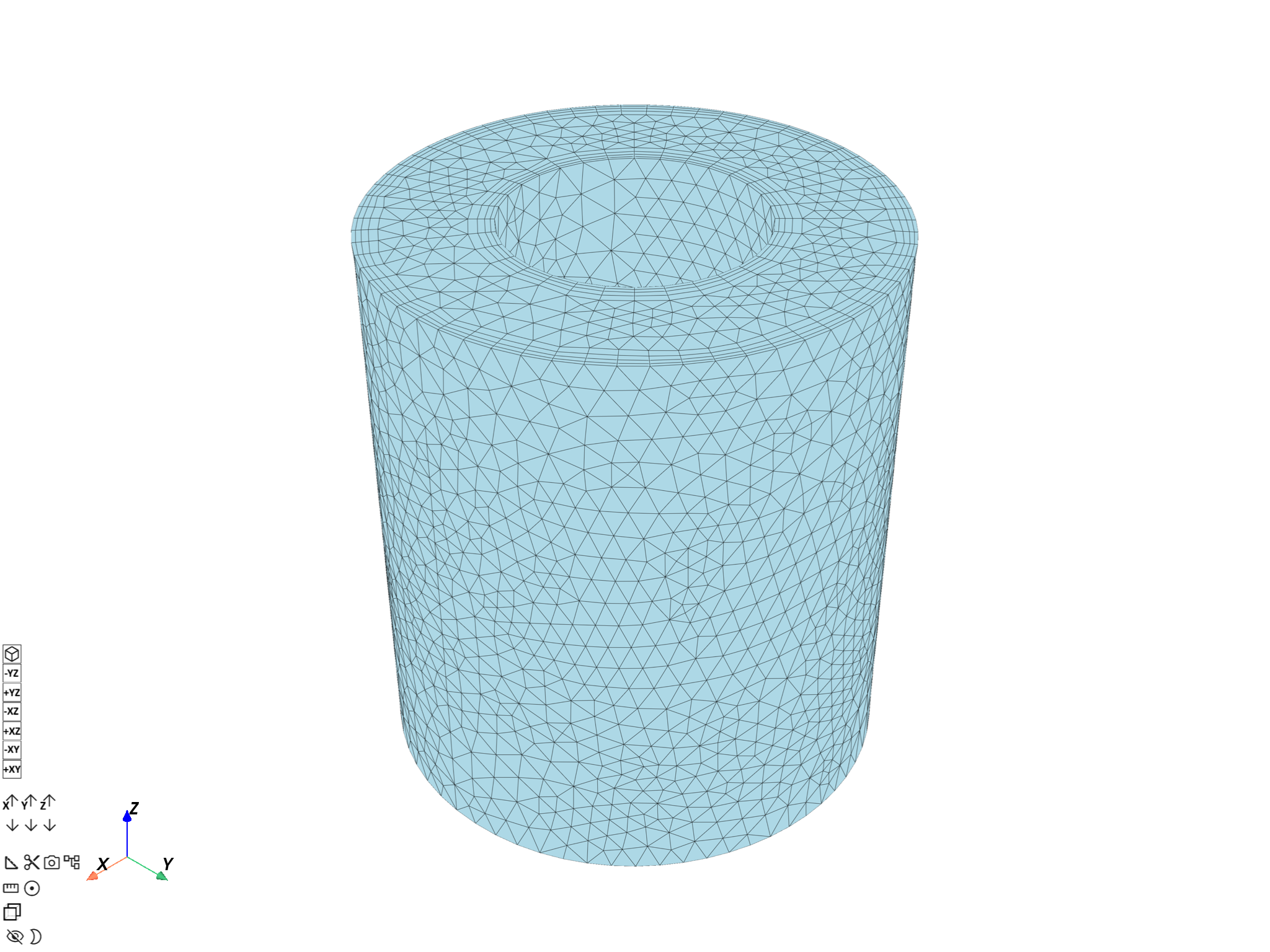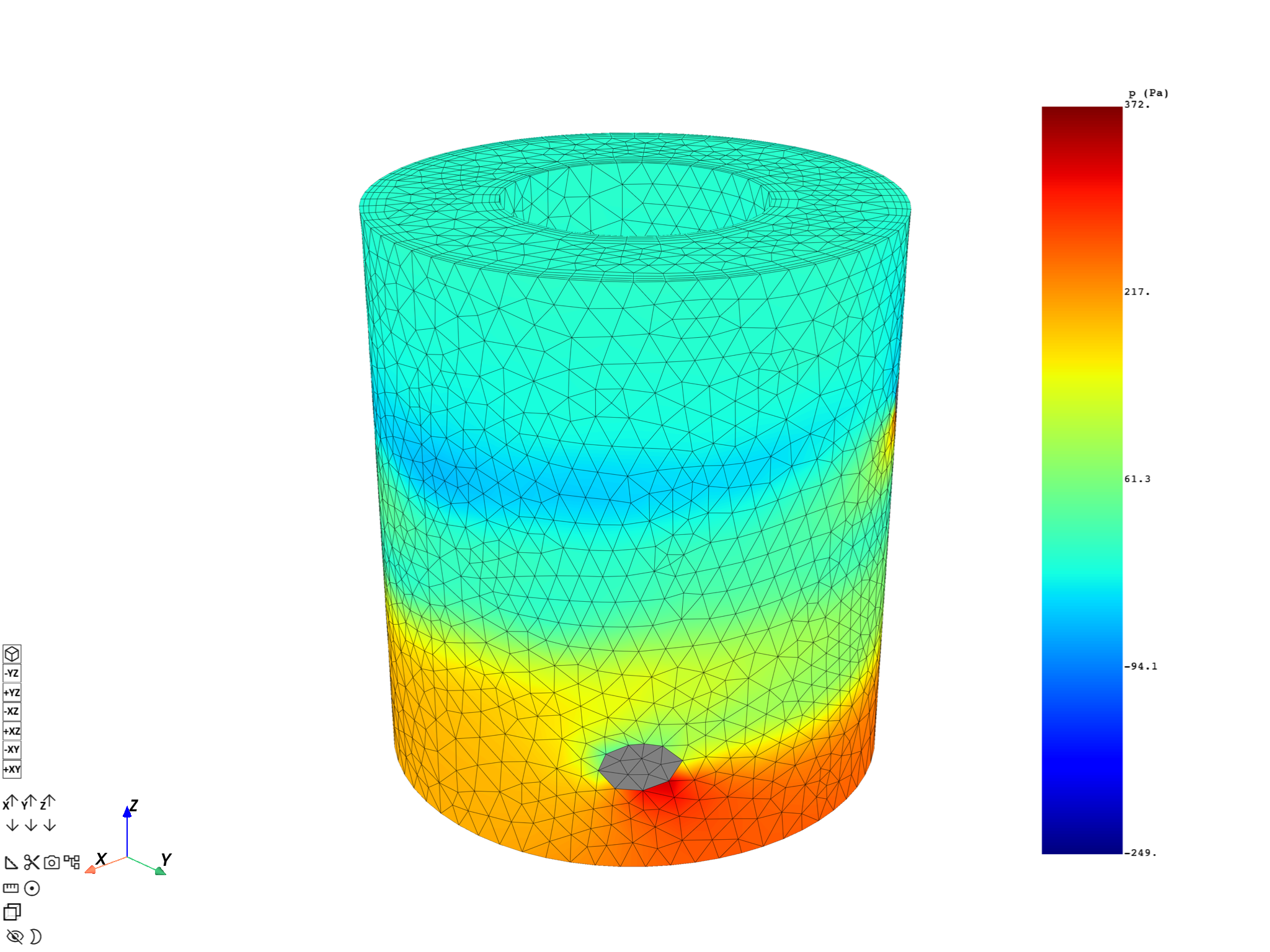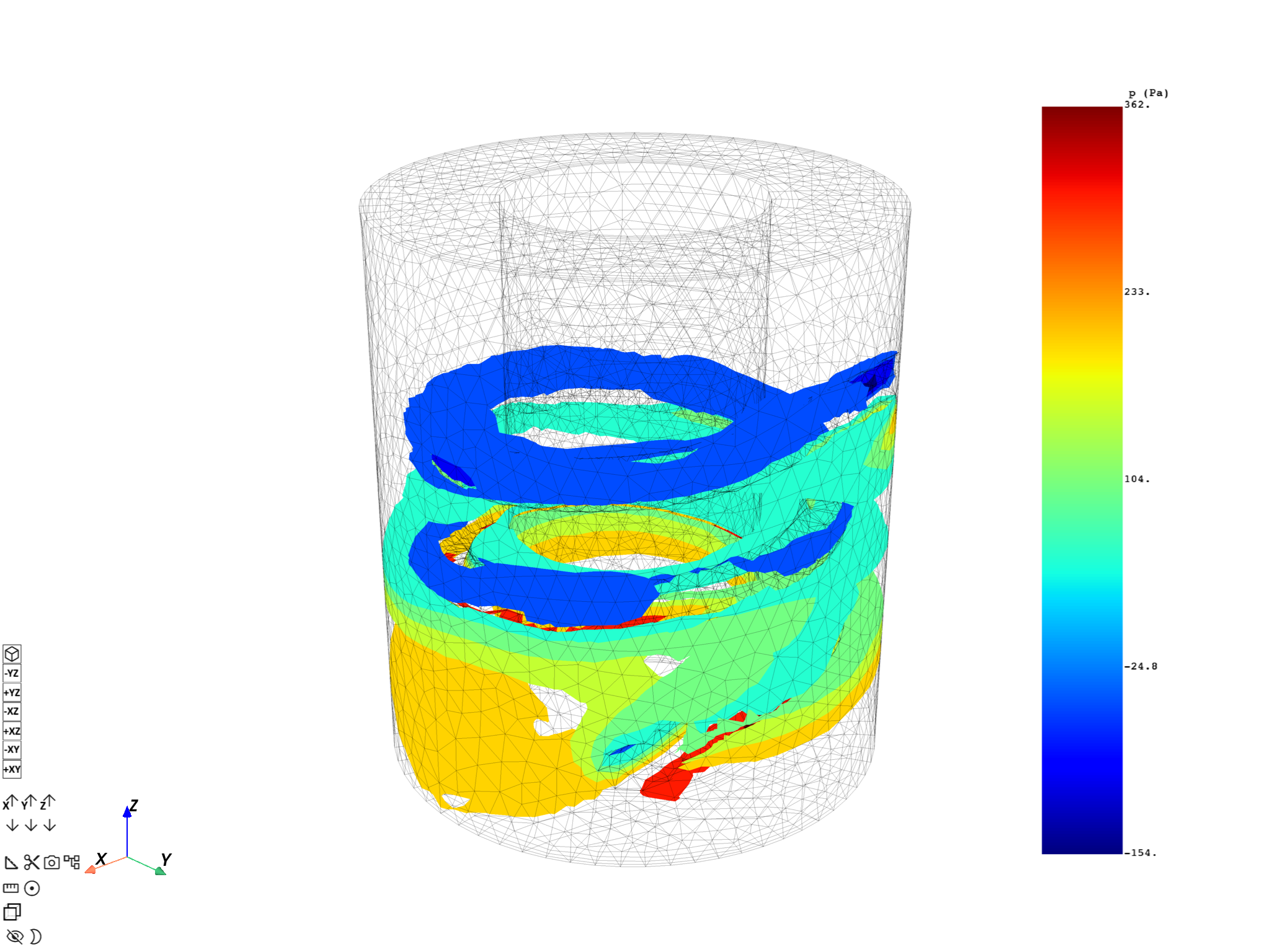# Compute iso-surfaces on fluid models#

This example demonstrates how to compute iso-surfaces on fluid models.

Note

This example requires DPF 7.0 (ansys-dpf-server-2024-1-pre0) or above. For more information, see Compatibility.

## Import the `dpf-core` module and its examples files.#

```import ansys.dpf.core as dpf
from ansys.dpf.core import examples
from ansys.dpf.core.plotter import DpfPlotter
```

## Specify the file path.#

We work on a cas/dat.h5 file with only nodal variables.

```path = examples.download_cfx_heating_coil()
ds = dpf.DataSources()
ds.set_result_file_path(path["cas"], "cas")
```

## Whole mesh scoping.#

We evaluate the mesh with the mesh_provider operator to scope the mesh_cut operator with the whole mesh.

```whole_mesh = dpf.operators.mesh.mesh_provider(streams_container=streams).eval()
print(whole_mesh)

whole_mesh.plot()
``````DPF  Meshed Region:
25456 nodes
74882 elements
Unit: m
With solid (3D) elements
```

## Extract the physics variable#

Here we choose to work with the static pressure by default which is a scalar and nodal variable without multi-species/phases. With a multi-species case, select one using qualifier ellipsis pins and connecting a LabelSpace “species”/”phase”.

```P_S = dpf.operators.result.static_pressure(streams_container=streams, mesh=whole_mesh).eval()
print(P_S)

pl = DpfPlotter()
cpos_mesh_variable = [
(4.256160478475664, 4.73662111240005, 4.00410065817644),
(-0.0011924505233764648, 1.8596649169921875e-05, 1.125),
(-0.2738679385987956, -0.30771426079547065, 0.9112125360807675),
]
pl.show_figure(cpos=cpos_mesh_variable, show_axes=True)
``````DPF p_<Mixture> Field
Location: Nodal
Unit: Pa
23648 entities
Data: 1 components and 23648 elementary data

IDs                   data(Pa)
------------          ----------
1                     8.211024e+01

2                     -4.502726e+01

3                     2.326057e+02

...
```

## Evaluate iso-surfaces#

We can finally use the mesh_cut operator on this specific variable. We choose to cut the whole with 5 iso-surface equally spaced between min and max.

```max_pressure = 361.8170  # Pa
min_pressure = -153.5356  # Pa
number_of_iso_surface = 5
step = (max_pressure - min_pressure) / number_of_iso_surface

pl = DpfPlotter()
c_pos_iso = [
(4.256160478475664, 4.73662111240005, 4.00410065817644),
(-0.0011924505233764648, 1.8596649169921875e-05, 1.125),
(-0.2738679385987956, -0.30771426079547065, 0.9112125360807675),
]
meshed_region=whole_mesh,
style="wireframe",
show_edges=True,
show_axes=True,
color="black",
opacity=0.3,
)

for i in range(number_of_iso_surface):
iso_surface = dpf.operators.mesh.mesh_cut(
field=P_S, iso_value=min_pressure, closed_surface=0, mesh=whole_mesh, slice_surfaces=True
).eval()
P_S_step = dpf.Field(location=dpf.locations.overall, nature=dpf.common.natures.scalar)
P_S_step.append([min_pressure], i)
P_S_step.name = "static pressure"
P_S_step.unit = "Pa"
field=P_S_step, meshed_region=iso_surface, style="surface", show_edges=False, show_axes=True
)
min_pressure += step

pl.show_figure(show_axes=True, cpos=c_pos_iso)
```# Important note#

Iso-surfaces computation through the mesh_cut operator are only supported for Nodal Fields. For Elemental variables, you must perform an averaging operation on the Nodes before running the mesh_cut operator. This can be done by chaining the elemental_to_nodal operator output with the mesh_cut operator input.

Total running time of the script: ( 0 minutes 7.099 seconds)

Gallery generated by Sphinx-Gallery